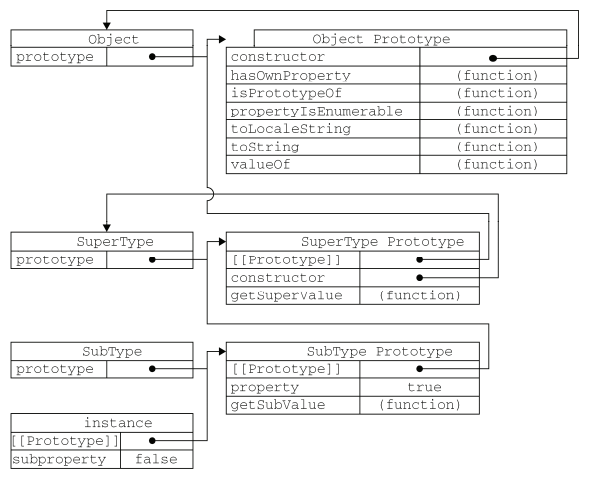﻿ JavaScript基于原型链的继承 - 鸿网互联

# JavaScript基于原型链的继承

Javascript并不是一门面向对象的语言，没有提供传统的继承方式，但是它提供了一种原型继承的方式，利用自身提供的原型属性来实现继承。

function SuperType() { this.property = true; } SuperType.prototype.getSuperValue = function () { return this.property; }; function SubType() { this.subproperty = false; } // 继承SuperType SubType.prototype = new SuperType(); SubType.prototype.getSubValue = function () { return this.subproperty; }; var instance = new SubType(); alert(instance.getSuperValue()); // true

SubType继承了SuperType,继承是通过创建SuperType的实例，并将该实例赋给SubType.prototype实现的。实现的本质是重写原型对象，换成一个新类型的实例。这样，原来存在于SuperType的实例中的属性和方法，也存在与SubType.prototype中了。然后给SubType.prototype添加一个方法，这样就继承了SuperType的属性和方法的基础上又添加了一个方法。function SuperType() { this.property = true; } SuperType.prototype.getSuperValue = function () { return this.property; }; function SubType() { this.subproperty = false; } SuperType.prototype = new SuperType(); // 添加方法 SubType.prototype.getSubValue = function () { return this.subproperty; }; // 覆盖超类中的方法 SubType.prototype.getSuperValue = function () { return false; }; var instance = new SubType(); alert(instance.getSuperValue()); // false

function SuperType() { this.property = true; } SuperType.prototype.getSuperValue = function () { return this.property; }; function SubType() { this.subproperty = false; } // 继承SuperType SubType.prototype = new SuperType(); // 使用字面量添加新方法，导致上一行代码无效 SubType.prototype = { getSubValue :function() { return this.subproperty; }, someOtherMethod: function () { return false; } }; var instance = new SubType(); alert(instance.getSuperValue()); // error

function SuperType() { this.colors = ["red", "blue", "green"]; } function SubType() { } SubType.prototype = new SuperType(); var instance1 = new SubType(); instance1.colors.push("black"); alert(instance1.colors); // "red", "blue", "green", "black" var instance2 = new SubType(); alert(instance2.colors); // "red", "blue", "green", "black"

<